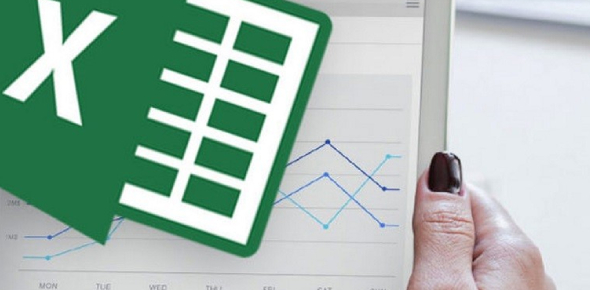# Microsoft Excel Quiz

20 Questions | Attempts: 127638
ShareSettingsDo you think you know the basics of Microsoft Excel very well? Take is Microsoft Excel quiz and test your knowledge! Have you just finished your training in Microsoft Excel and are looking to test out your understanding? The practice test below is set to test how well you can use excel effectively. Give it a try and ensure you read up on the parts you don't get correctly. Along with practice, you will learn new things too. If you find this quiz a good one and informative, share it with others also.

• 1.
A fast way to add up the column of numbers is to click on the cell below the numbers and then:
• A.

Click Subtotals on the Data menu.

• B.

View the sum in the formula bar.

• C.

Click the AutoSum button on the Standard toolbar, then press ENTER.

• D.

Click the Sum button on the Standard toolbar.

• 2.
If you want to paste a formula result — but not the underlying formula — to another cell. You would copy the cell with the formula, then place the insertion point in the cell you want to copy to. What next?
• A.

Click the Paste button on the Standard toolbar.

• B.

Click the arrow on the Paste button on the Standard toolbar, then click Formulas.

• C.

Click the arrow on the Paste button on the Standard toolbar, then click Values.

• D.

Click the arrow on the Copy button on the Standard toolbar.

• 3.
How do you change the column width to fit the contents?
• A.

Single-click the boundary to the left of the column heading.

• B.

Double-click the boundary to the right of the column heading.

• C.

Press ALT and single-click anywhere in the column.

• D.

Press Fn and double-click anywhere in the column.

• 4.
There are three worksheets with every new workbook. You can change that automatic number if you want to.
• A.

True

• B.

False

• 5.
###### means:
• A.

You've entered a number wrong.

• B.

You've misspelled something.

• C.

The cell is not wide enough.

• D.

The cell has the wrong data.

• 6.
To add a new row, click a cell in the row immediately above where you want the new row.
• A.

True

• B.

False

• 7.
Which key do you press to group two or more nonadjacent worksheets?
• A.

CTRL

• B.

SHIFT

• C.

ALT

• D.

TAB

• 8.
To copy an entire worksheet and all its data, you should click the worksheet tab of the sheet that you want to copy, hold down SHIFT, and then drag the selected sheet along the row of sheet tabs.
• A.

True

• B.

False

• 9.
A user wishes to remove a spreadsheet from a workbook. Which is the correct sequence of events that will do this?
• A.

Go to FILE - SAVE AS - SAVE AS TYPE - Excel 4.0 Work Sheet

• B.

Right-click on the spreadsheet tab and select DELETE.

• C.

Right-click on the spreadsheet and select INSERT - ENTIRE COLUMN.

• D.

Left-click on the spreadsheet and select SAVE.

• 10.
Which formula can add all the numeric values in a range of cells, ignoring those which are not numeric, and place the result in a different cell?
• A.

Count

• B.

Average

• C.

Sum

• D.

• 11.
Is it possible to insert an image from a file into an Excel spreadsheet?
• A.

Yes

• B.

No

• 12.
Can an Excel spreadsheet be used as the "data source" for a Word Mail Merge?
• A.

Yes

• B.

No

• 13.
On an Excel sheet, the active cell is indicated by ____.
• A.

A wide dark border

• B.

A dotted border

• C.

• D.

None of the above

• 14.
Formula and function are the same things.
• A.

True

• B.

False

• 15.
In order to multiple items in Excel, you would use:
• A.

^

• B.

@

• C.

*

• D.

#

• 16.
The formula = ((A2+B5)*5% is valid.
• A.

True

• B.

False

• 17.
If cells: A1=90 A2=85 A3=80 A4=75 A5=75 What will be your formula if you are going to get the average?
• 18.
If cells: A1=90 A2=85 A3=80 A4=75 A5=75 What will be your formula if you are going to get the total?
• 19.
Get the remarks in cell E10 that valued 75, which noted that if the remarks are higher than 74, the remarks will be "PASSED" or else "FAILED."
• 20.
Get the remarks in cell A1 that valued 75, which noted that if the remarks are higher than 74, the remarks will be "PASSED" or else "FAILED".

## Related TopicsBack to top
×

Wait!
Here's an interesting quiz for you.